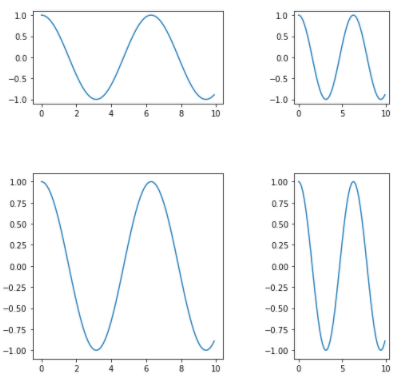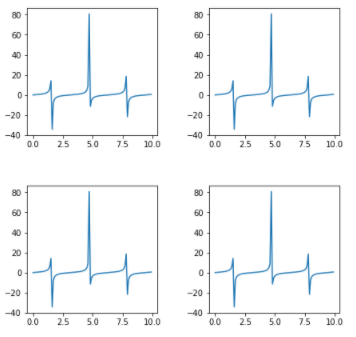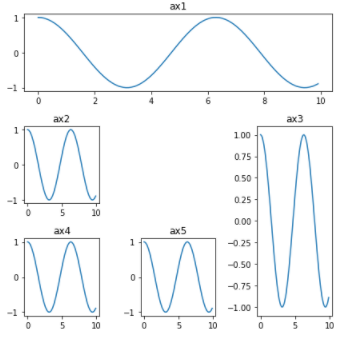# How to Create Different Subplot Sizes in Matplotlib?

• Difficulty Level : Medium
• Last Updated : 23 Sep, 2021

In this article, we will learn different ways to create subplots of different sizes using Matplotlib. It provides 3 different methods using which we can create different subplot of different sizes.

Methods available to create subplot :

• Gridspec
• gridspec_kw
• subplot2grid

1. Gridspec : GridSpec from the gridspec module used to adjust the geometry of Subplot grid. We can use different parameters to adjust shape, size, no. of columns and rows.

Code :

## Python3

 `# importing required libraries``import` `matplotlib.pyplot as plt``from` `matplotlib ``import` `gridspec``import` `numpy as np` `# create a figure``fig ``=` `plt.figure()` `# to change size of subplot's``# set height of each subplot as 8``fig.set_figheight(``8``)` `# set width of each subplot as 8``fig.set_figwidth(``8``)` `# create grid for different subplots``spec ``=` `gridspec.GridSpec(ncols``=``2``, nrows``=``2``,``                         ``width_ratios``=``[``2``, ``1``], wspace``=``0.5``,``                         ``hspace``=``0.5``, height_ratios``=``[``1``, ``2``])` `# initializing x,y axis value``x ``=` `np.arange(``0``, ``10``, ``0.1``)``y ``=` `np.cos(x)` `# ax0 will take 0th position in``# geometry(Grid we created for subplots),``# as we defined the position as "spec"``ax0 ``=` `fig.add_subplot(spec[``0``])``ax0.plot(x, y)` `# ax1 will take 0th position in``# geometry(Grid we created for subplots),``# as we defined the position as "spec"``ax1 ``=` `fig.add_subplot(spec[``1``])``ax1.plot(x, y)` `# ax2 will take 0th position in``# geometry(Grid we created for subplots),``# as we defined the position as "spec"``ax2 ``=` `fig.add_subplot(spec[``2``])``ax2.plot(x, y)` `# ax3 will take 0th position in``# geometry(Grid we created for subplots),``# as we defined the position as "spec"``ax3 ``=` `fig.add_subplot(spec[``3``])``ax3.plot(x, y)` `# display the plots``plt.show()`

Output :Explanation :

# create grid for different subplots
spec = gridspec.GridSpec(ncols=2, nrows=2, width_ratios=[2, 1],wspace=0.5,hspace=0.5,height_ratios=[1,2])

Here “gridspec.GridSpec()” will create grids for subplots.We can use different parameters to adjust the grid and each plot size.

• ncols : pass number of columns you want in Grid.
• nrows : pass number of rows we want in Grid to make subplots.
• width_ratios : set width ratio of subplot(adjust the width of plot).
• height_ratios : set height ratio of subplot(adjust the height of plot).
• wspace : give “wspace” amount of space vertically to separate the subplots.
• hspace : give “hspace” amount of space horizontally to separate the subplots.

2. gridspec_kw : It is a dictionary available inside “plt.subplots()” method in Matplotlib. By passing different parameters to the dictionary we can adjust the shape and size of each subplot.

Code :

## Python3

 `# importing required libraries``import` `matplotlib.pyplot as plt``import` `numpy as np` `# setting different parameters to adjust each grid``fig, ax ``=` `plt.subplots(nrows``=``2``, ncols``=``2``, figsize``=``(``7``, ``7``),``                       ``gridspec_kw``=``{``                           ``'width_ratios'``: [``3``, ``3``],``                           ``'height_ratios'``: [``3``, ``3``],``                       ``'wspace'``: ``0.4``,``                       ``'hspace'``: ``0.4``})`  `# initializing x,y axis value``x ``=` `np.arange(``0``, ``10``, ``0.1``)``y ``=` `np.tan(x)` `# ax will take 0th position in``# geometry(Grid we created for subplots)``ax[``0``][``0``].plot(x, y)` `# ax will take 0th position in``# geometry(Grid we created for subplots)``ax[``0``][``1``].plot(x, y)` `# ax will take 0th position in``# geometry(Grid we created for subplots)``ax[``1``][``0``].plot(x, y)` `# ax will take 0th position in``# geometry(Grid we created for subplots)``ax[``1``][``1``].plot(x, y)` `plt.show()`

Output :Explanation :

# setting different parameters to adjust each grid
fig, ax = plt.subplots(nrows=2, ncols=2,figsize=(7,7), gridspec_kw={‘width_ratios’: [3, 3], ‘height_ratios’: [3, 3], ‘wspace’ : 0.4, ‘hspace’ : 0.4})

“gridspec_kw = {}” is dictionary with keywards using which we can change shape, size and adjust each grid.

• nrows : number of rows in grid
• ncols : number of columns in grid
• width_ratios : set width size of each subplot
• height_ratios : set height size of each subplot
• wspace : give “wspace” amount of space vertically to separate the subplots.
• hspace : give “hspace” amount of space horizontally to separate the subplots.
• figsize : set size of subplot’s.

3. subplot2grid : It provides more flexibility to create a grid at any location. We can extend the grid horizontally as well as vertically very easily.

Code :

## Python3

 `# importing required library``import` `matplotlib.pyplot as plt``import` `numpy as np` `# creating grid for subplots``fig ``=` `plt.figure()``fig.set_figheight(``6``)``fig.set_figwidth(``6``)` `ax1 ``=` `plt.subplot2grid(shape``=``(``3``, ``3``), loc``=``(``0``, ``0``), colspan``=``3``)``ax2 ``=` `plt.subplot2grid(shape``=``(``3``, ``3``), loc``=``(``1``, ``0``), colspan``=``1``)``ax3 ``=` `plt.subplot2grid(shape``=``(``3``, ``3``), loc``=``(``1``, ``2``), rowspan``=``2``)``ax4 ``=` `plt.subplot2grid((``3``, ``3``), (``2``, ``0``))``ax5 ``=` `plt.subplot2grid((``3``, ``3``), (``2``, ``1``), colspan``=``1``)`  `# initializing x,y axis value``x ``=` `np.arange(``0``, ``10``, ``0.1``)``y ``=` `np.cos(x)` `# plotting subplots``ax1.plot(x, y)``ax1.set_title(``'ax1'``)``ax2.plot(x, y)``ax2.set_title(``'ax2'``)``ax3.plot(x, y)``ax3.set_title(``'ax3'``)``ax4.plot(x, y)``ax4.set_title(``'ax4'``)``ax5.plot(x, y)``ax5.set_title(``'ax5'``)` `# automatically adjust padding horizontally``# as well as vertically.``plt.tight_layout()` `# display plot``plt.show()`

Output :Explanation :

ax1 = plt.subplot2grid(shape=(3, 3), loc=(0, 0), colspan=3)
ax2 = plt.subplot2grid(shape=(3, 3), loc=(1, 0), colspan=1)
ax3 = plt.subplot2grid(shape=(3, 3), loc=(1, 2), rowspan=2)
ax4 = plt.subplot2grid((3, 3), (2, 0))
ax5 = plt.subplot2grid((3, 3), (2, 1),colspan=1)

Here shape is denoting to no. of rows and columns and loc denotes to geometric location of grid. Suppose if we are using colspan = 3 in ax1,it means these subplot will cover 3 all columns of that row. Similarly in ax2,colspan=1 means it will cover 1 column space at its location. In case of ax3,rowspan=2 means it will cover space of 2 rows.If we give irregular input then it will give error so we have give proper value to colspan and rowspan.

My Personal Notes arrow_drop_up